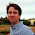### Fibonacci Multiple Puzzle

Source: Mailed to me by Kushagra Singhal, Ex-IIT Kanpur, PhD Student at University of Illinois at Urbana-Champaign

Problem:

Prove that for any positive K and a natural number n, every (n*K)th number in the Fibonacci sequence is a multiple of the Kth number in the Fibonacci sequence.

More formally, for any natural number n, let F(n) denote Fibonacci number n. That is, F(0) = 0, F(1) = 1, and F(n+2) = F(n+1) + F(n). Prove that for any positive K and natural n, F(n*K) is a multiple of F(K).

1.Lemma. F(n) = F(k+1).F(n-k) + F(k).F(n-k-1)
Proof.
F(n)
= F(2).F(n-1) + F(1).F(n-2)
= F(3).F(n-2) + F(2).F(n-3)
= ...
= F(k+1).F(n-k) + F(k).F(n-k-1)

Now for proof of the main question, suppose F(k) divides F((n-1)k) [inductive hypothesis].
From above lemma, we have,
F(n.k) = F(k+1).F((n-1).k) + F(k).F((n-1)k - 1)
Since F(k) divides F((n-1)k), F(k) divides F(n.k).
QED

2.Use the formula for F_n given here. https://www.math.hmc.edu/funfacts/ffiles/10002.4-5.shtml

Also, a-b divides a^n-b^n. Let a=Phi^k and b=phi^k. Observe that the factor (a^n-b^n)/(a-b) is an integer.

3.We prove this using induction. Before that, we make a small observation about Fibonacci numbers

For any positive integer k,
F(k+2) = F(k+1) + F(k)
F(k+3) = 2*F(k+1) + F(k)
F(K+4) = 3*F(k+1) + 2*F(k)
...
F(k+m) = F(m)*F(k+1) + F(m-1)*F(k)
where m is a positive integer

We need to prove that for a positive integers K and n, F(n*K) = c*F(K) where c is a positive integer.
Setting K to be any positive integer, and applying induction on n,
The base case is n = 1. F(K) = 1*F(K). Thus the base case holds.
Assuming the statement is true for some n. F(n*K) = c*F(K)
Now,
F((n+1)*K) = F(n*K+K) = F(K)*F(n*K+1) + F(K-1)*F(n*K)
But F(n*K) = c*F(K).
Thus F((n+1)*K) = F(K)*(F(n*K+1)+c*F(K-1)) = c'*F(K) where c' is a positive integer
Thus the induction holds.

The given statement is true for all positive integers K and n.

4.Lemma:
For 0 <= k < n:
F(n) = F(k+1) * F(n-k) + F(k) * F(n-k-1)

This can be proven by induction on k.
base case k = 0:
F(n) = 1 * F(n) + 0 * F(n-1)

induction:
Since F(n-k) = F(n-k-1) + F(n-k-2), one can rearrange coefficients, so that
F(n) = F(k+1) * F(n-k) + F(k) * F(n-k-1)
F(n) = F(k+1) * (F(n-k-1) + F(n-k-2)) + F(k) * F(n-k-1)
F(n) = (F(k+1) + F(k)) * F(n-k-1) + F(k+1) * F(n-k-2)
F(n) = F(k+2) * F(n-k-1) + F(k+1) * F(n-k-2)

To finish, use induction on n to show that F(n*K) is a multiple of F(K).
base case n = 0:
F(0) = 0 is a multiple of F(K)

induction:
By the lemma,
F((n+1)*K) = F(K+1) * F(n*K) + F(K) * F(n*K-1)
and since both F(n*K) and F(K) are multiple of K, so is F((n+1)*K)

5.Here is a brute force method:

its well known that F(n)= [a^n-b^n]/sqrt (5) where a= (1+sqrt(5))/2 and b= (1-sqrt(5))/2.
so its required to prove that [a^(n*k)-b^(n*k)]/(a^k-b^k) is a whole number.

in fact a little algebra shows that above expression is a whole number for natural n and k ,and real numbers a and b , provided a+b and a*b are whole numbers.

outline of proof:
let x=a^k and y=b^k.
prove by induction , that x^i+y^i is a whole number for any natural i.
its clear that for natural i , (x*y)^i is also a whole number as a*b is a whole number.

expression of interest = [x^n-y^n]/[x-y].
=sigma (x^i)* y^(n-1-i), i = 0 to n-1.
if n is even, the expression equals sigma (x*y)^i * [ (x^(n-1-2*i)+y^(n-1-2*i) ], i = 0 to (n-2)/2
if n is odd , the expression equals (x*y)^((n-1)/2) +sigma (x*y)^i * [ (x^(n-1-2*i)+y^(n-1-2*i) ], i = 0 to (n-3)/2.

hence proved as (x*y)^i and x^i+y^i are whole numbers for all natural numbers i.

6.Assume that F(nK) is a multiple of F(K). We shall prove that F((n+1)K) is also a multiple of F(K).

F(nK+2) = F(nK+1) + F(nK)
F(nK+3) = F(nK+2) + F(nK+1) = 2F(nK+1) + F(nK)
F(nK+4) = F(nK+3) + F(nK+2) = 3F(nK+1) + 2F(nK)
F(nK+5) = F(nK+4) + F(nK+3) = 5F(nK+1) + 3F(nK)
F(nK+6) = F(nK+5) + F(nK+4) = 8F(nK+1) + 5F(nK)
.
.
.
F(nK+i) = F(i) F(nK+1) + F(i-1) F(nK)

Thus, when i=K, we have F((n+1)K) = F(K) F(nK+1) + F(K-1) F(nK), which is a multiple of F(K) as both terms are multiples of F(K) (recall that F(nK) is a multiple of F(K)).

7.F(n+1) = F(n-1)+F(n)
F(n+2) = F(n-1)+2F(n)
F(n+3)= 2F(n-1)+3F(n)
.
.
.
F(n+k)=F(k)F(n-1)+F(k+1)F(n) -- 1
so, F(2n) = F(n)F(n-1)+F(n+1)F(n) = t*F(n)
induction:
assume F(nk) = L*F(n) ( since we proved F(2n) = t*F(n))
F(n(k+1)) = F(nk+n)=F(n)F(nk-1)+F(n+1)(F(nk) = L*F(n))
rhs is divisible by F(n)

8.Any fibonacci number could be written as sum of any two (lower) value of fibonacci numbers. for example:

f(10) = f(9) + f(8)
= 2f(8) + f(7)
= 3f(7) + 2f(6)
and so on..

So, if we split f(nk) it could be written as

f(nk) = x1*f(k+1) + x2*f(k)

These coefficients also follows fibonacci pattern. For example:

f(10) = f(2)*f(10-1) + f(1)*f(10-2)
= f(3)*f(10-2) + f(2)*f(10-3)
= f(4)*f(10-3) + f(3)*f(10-4)
and so on...

therefore x1 will be equals to f((n-1)k)

now the equation could be written as:

f(nk) = f((n-1)k)*f(k+1) + x2*f(k)

which could be re-written as

f(nk) = [ f((n-1)k) ] *f(k+1) + x2*f(k)
= [ x3*f(k+1) + x4*f(k) ] * f(k+1) + x2*f(k)
= [ f((n-2)k)*f(k+1) + x4*f(k) ] * f(k+1) + x2*f(k)

and so on...
and the final equation would be like:

f(nk) = f(k)*a1*f(k+1) + a2*f(k)
= f(k) [ a1*f(k+1) + a2 ]

and at one point the coefficient of f(k+1) will be a multiple of f(k).
Hence every f(nk) has f(k) as its factor.